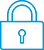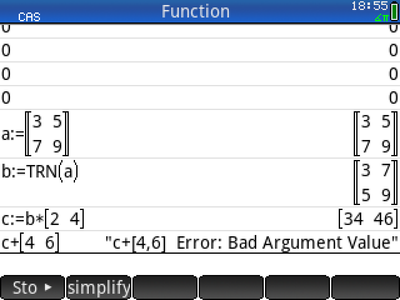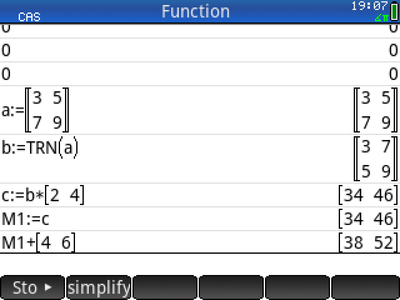cancel
Showing results for
Search instead for
Did you mean:It has been a while since anyone has replied. Simply ask a new question if you would like to start the discussion again.New member
5 2 0 0
Message 1 of 4
622
Flag Post

# Problems with local variables in Cas programsHP Recommended
HP Prime

Hello everyone,

I have trouble with my CAS program, it seems there's a problem with some local variables.

This is the program:

#cas
newmult(varx):=
BEGIN
LOCAL varv,xvnm,jac,fun,jacev,funev;
LOCAL J,L,M,M1,M2,M3,M4;
varv:=[varx];
M3:=varv(3);
M:=SIZE(varv(3));
L:=varv(4);
M1:=[];
REDIM(M1,{L+1,M});
M4:=;
REDIM(M4,L+1);
M1(1):=M3;
xvnm:=varv(2)=varv(3);
jac:=TRN(diff(varv(1),varv(2)));
fun:=varv(1);
FOR J FROM 1 TO L DO
jacev:=subst(jac,xvnm);
funev:=subst(fun,xvnm);

//IT SEEMS THE PROBLEM LIES IN THE NEXT LINE
M2:=−1*(jacev^(−1))*funev;

M3:=M3+M2;
M1(J+1):=M3;
M4(J+1):=l2norm(M2);
xvnm:=varv(2)=M3;
END;
ADDCOL(M1,M4,M+1);
EDITMAT(M1,"RESULTS");
return jac;
END;
#end

So the problem is this:

M2:=−1*(jacev^(−1))*funev;

The input should be something like this:

([x^2-10*x+y^2+8 , x*y^2+x-10*y+8],[x, y],[0,0],4)

I deleted command LOCAL and the program worked well, however when I use the command LOCAL an error message appears ("Error: Bad Argument Value"). Also, when I delete only M2 in the Local command, the program works well; that means that there's no problem when M2 is a global variable.

I don't want to delete the LOCAL command neither make M2 a global variable. So, what's the problem or what should I do?

I have some other questions about the CAS programs like: Is it possible to use the IF command or the IFERR command in the CAS programs? How shoild I use them? What commands are not allowed in these programs?

Thanks for your answers.

Tags (3)
3 REPLIES 3Level 6
305 297 32 76
Message 2 of 4
Flag PostHP Recommended

Hello dj2631,

Can you tell us what your input is?

It is difficult to find a bug without knowing what the input is!

It would even better if you said something about what the program is supposed to do.

Without knowing these things I think I see a problem though.

You write:

varv:=[varx];
M3:=varv(3);

So varv has only 1 element, namely varx.

So varv(3) does not exist and will cause an error.New member
5 2 0 0
Message 3 of 4
Flag PostHP Recommended

The input should be something like this:

([x^2-10*x+y^2+8 , x*y^2+x-10*y+8],[x, y],[0,0],4)

The only problem here is the LOCAL VARIABLE M2:
If define M2 as a LOCAL VARIABLE, an error mesage appears:
"Error: Bad Argument Value"
If I use M2 as GLOBAL VARIABLE the program works great.Level 6
305 297 32 76
Message 4 of 4
Flag PostHP Recommended

In my opinion there are 2 causes of problems with this input.

First I think there is a bug in the TRN command.

Second, you are doing exact calculations in CAS, which means that the matrix elements have forms like

(9234543567854356754323454350/2345676567896545673456543445656788)*SQRT(22345678987432456765436784324567654567)

After a number of loops the integers are immense, which causes an error.

You can easily prevent this by unchecking  the exact box in CAS settings or by entering [0.0, 0.0] (so decimal numbers) as third argument.

Or by using approx() somewhere in the program.

I said I think that there is a bug in the TRN command.

See this:So I think there is something wrong with the type of b and c.

This can be corrected by storing b or c into the  global Home matrix M1:Or:There are also other ways to correct this, without using the global Home matrix M1.

When you are interested I can say something about this in another message.

You can verify that the problem is caused by the matrix jac in your program by replacing it by the matrix you know it should be.

When you do this you will probably see that the program works well.

† The opinions expressed above are the personal opinions of the authors, not of HP. By using this site, you accept the Terms of Use and Rules of Participation